## Sinusoidal Response of RL Circuit:

Consider a Sinusoidal Response of RL Circuit consisting of resistance and inductance as shown in Fig. 12.16.The switch, S, is closed at t = 0. At t = 0, a sinusoidal voltage V cos (ωt + θ) is applied to the series R-L circuit, where V is the amplitude of the wave and θ is the phase angle. Application of Kirchhoff’s voltage law to the Sinusoidal Response of RL Circuit results in the following differential equation.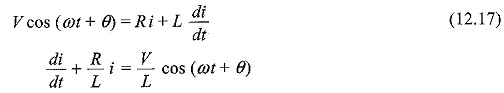The corresponding characteristic equation is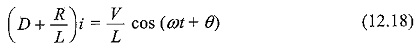For the above equation, the solution consists of two parts, viz. complementary function and particular integral.

The complementary function of the solution i is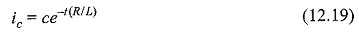The particular solution can be obtained by using undetermined co-efficients.

By assuming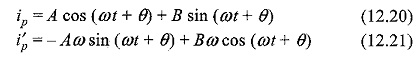Substituting Eqs 12.20 and 12.21 in Eq. 12.18, we have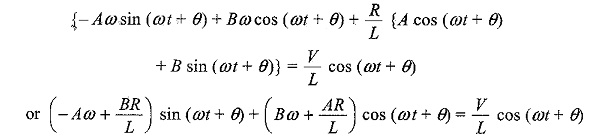Comparing cosine terms and sine terms, we get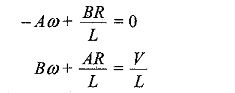From the above equations, we have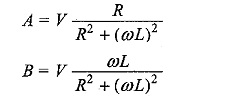Substituting the values of A and B in Eq. 12.20, we get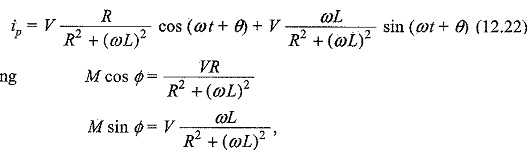to find M and Φ, we divide one equation by the other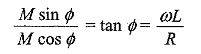Squaring both equations and adding, we get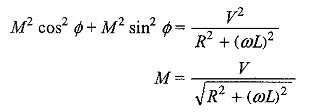The particular current becomes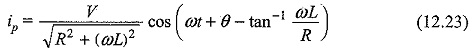The complete solution for the current i = ic + ip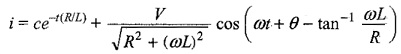Since the inductor does not allow sudden changes in currents, at t = 0, i = 0The complete solution for the current is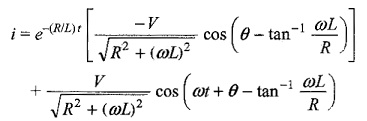Scroll to Top